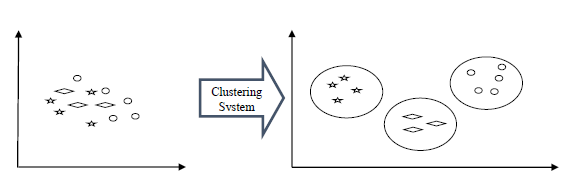# Clustering Algorithms - Overview

## Introduction to Clustering

Clustering methods are one of the most useful unsupervised ML methods. These methods are used to find similarity as well as the relationship patterns among data samples and then cluster those samples into groups having similarity based on features.

Clustering is important because it determines the intrinsic grouping among the present unlabeled data. They basically make some assumptions about data points to constitute their similarity. Each assumption will construct different but equally valid clusters.

For example, below is the diagram which shows clustering system grouped together the similar kind of data in different clusters −## Cluster Formation Methods

It is not necessary that clusters will be formed in spherical form. Followings are some other cluster formation methods −

### Density-based

In these methods, the clusters are formed as the dense region. The advantage of these methods is that they have good accuracy as well as good ability to merge two clusters. Ex. Density-Based Spatial Clustering of Applications with Noise (DBSCAN), Ordering Points to identify Clustering structure (OPTICS) etc.

### Hierarchical-based

In these methods, the clusters are formed as a tree type structure based on the hierarchy. They have two categories namely, Agglomerative (Bottom up approach) and Divisive (Top down approach). Ex. Clustering using Representatives (CURE), Balanced iterative Reducing Clustering using Hierarchies (BIRCH) etc.

### Partitioning

In these methods, the clusters are formed by portioning the objects into k clusters. Number of clusters will be equal to the number of partitions. Ex. K-means, Clustering Large Applications based upon randomized Search (CLARANS).

### Grid

In these methods, the clusters are formed as a grid like structure. The advantage of these methods is that all the clustering operation done on these grids are fast and independent of the number of data objects. Ex. Statistical Information Grid (STING), Clustering in Quest (CLIQUE).

## Measuring Clustering Performance

One of the most important consideration regarding ML model is assessing its performance or you can say model’s quality. In case of supervised learning algorithms, assessing the quality of our model is easy because we already have labels for every example.

On the other hand, in case of unsupervised learning algorithms we are not that much blessed because we deal with unlabeled data. But still we have some metrics that give the practitioner an insight about the happening of change in clusters depending on algorithm.

Before we deep dive into such metrics, we must understand that these metrics only evaluates the comparative performance of models against each other rather than measuring the validity of the model’s prediction. Followings are some of the metrics that we can deploy on clustering algorithms to measure the quality of model −

## Silhouette Analysis

Silhouette analysis used to check the quality of clustering model by measuring the distance between the clusters. It basically provides us a way to assess the parameters like number of clusters with the help of Silhouette score. This score measures how close each point in one cluster is to points in the neighboring clusters.

## Types of ML Clustering Algorithms

The following are the most important and useful ML clustering algorithms −

### K-means Clustering

This clustering algorithm computes the centroids and iterates until we it finds optimal centroid. It assumes that the number of clusters are already known. It is also called flat clustering algorithm. The number of clusters identified from data by algorithm is represented by ‘K’ in K-means.

### Mean-Shift Algorithm

It is another powerful clustering algorithm used in unsupervised learning. Unlike K-means clustering, it does not make any assumptions hence it is a non-parametric algorithm.

### Hierarchical Clustering

It is another unsupervised learning algorithm that is used to group together the unlabeled data points having similar characteristics.

We will be discussing all these algorithms in detail in the upcoming chapters.

## Applications of Clustering

We can find clustering useful in the following areas −

Data summarization and compression − Clustering is widely used in the areas where we require data summarization, compression and reduction as well. The examples are image processing and vector quantization.

Collaborative systems and customer segmentation − Since clustering can be used to find similar products or same kind of users, it can be used in the area of collaborative systems and customer segmentation.

Serve as a key intermediate step for other data mining tasks − Cluster analysis can generate a compact summary of data for classification, testing, hypothesis generation; hence, it serves as a key intermediate step for other data mining tasks also.

Trend detection in dynamic data − Clustering can also be used for trend detection in dynamic data by making various clusters of similar trends.

Social network analysis − Clustering can be used in social network analysis. The examples are generating sequences in images, videos or audios.

Biological data analysis − Clustering can also be used to make clusters of images, videos hence it can successfully be used in biological data analysis.